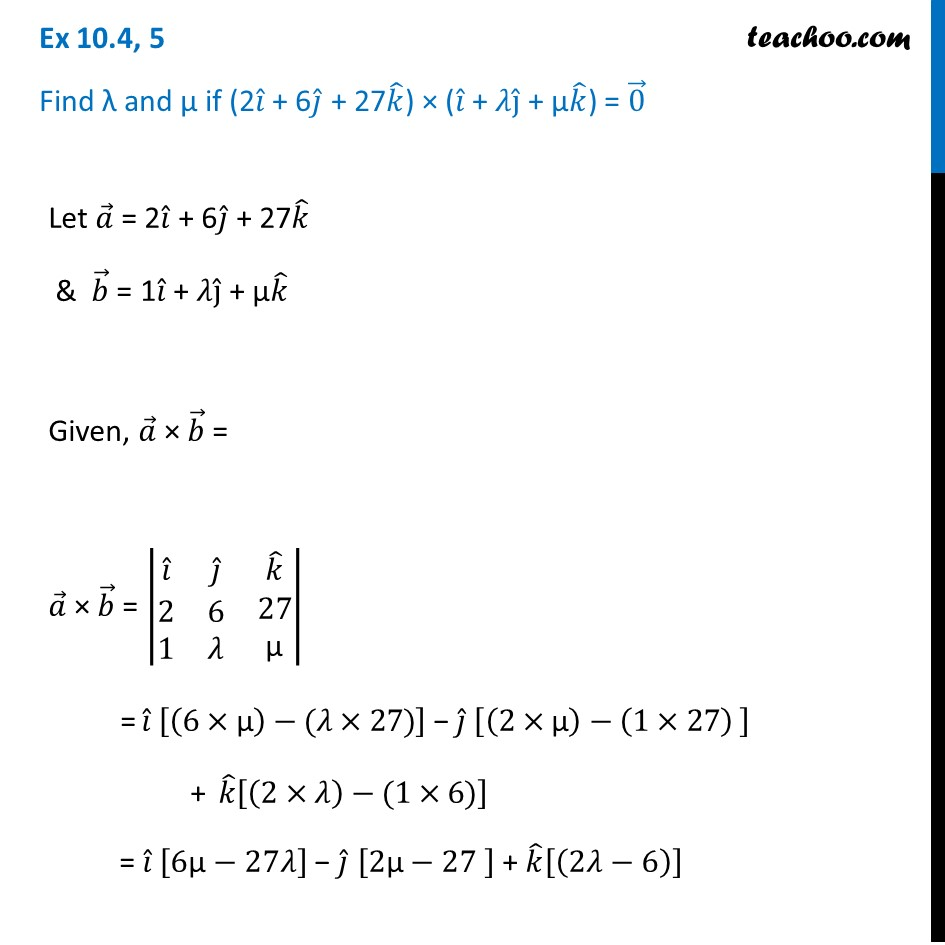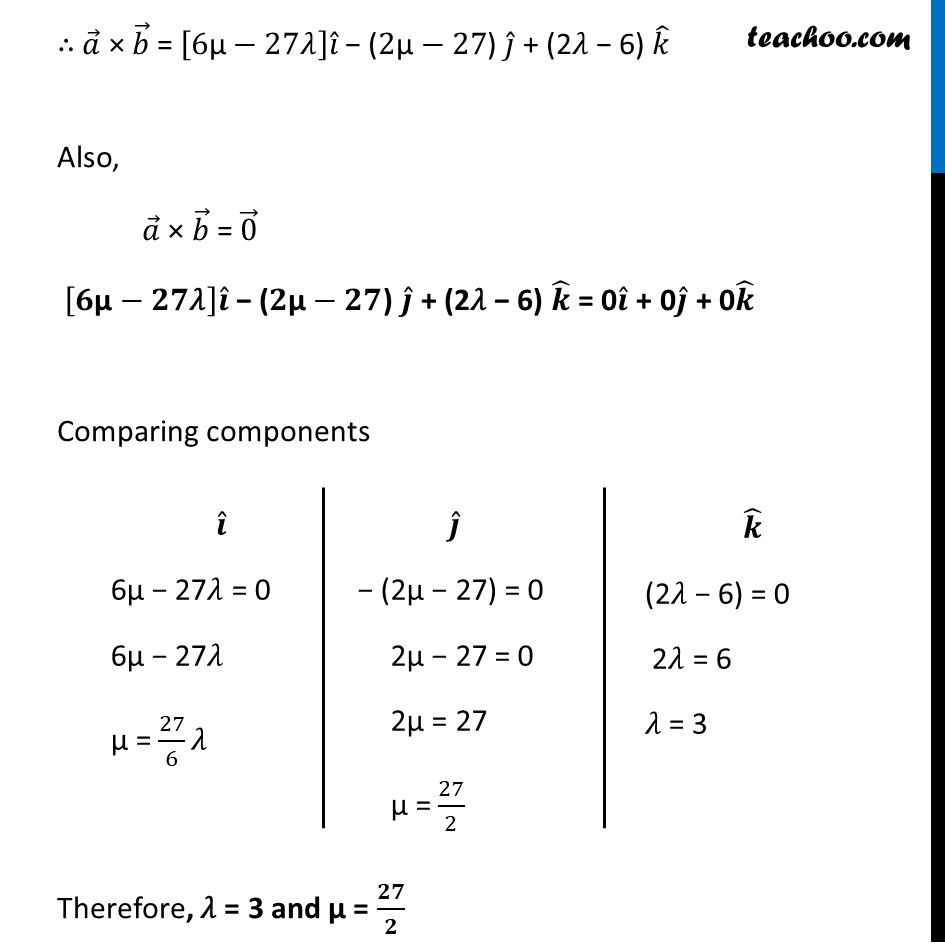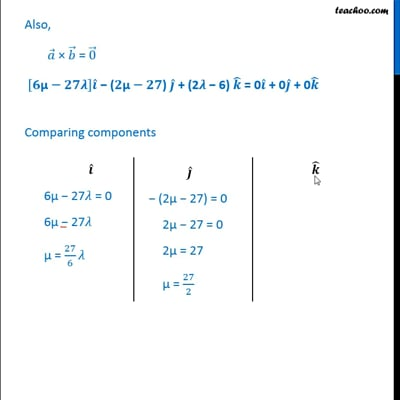Vector product - Defination

Chapter 10 Class 12 Vector Algebra
Concept wiseThis video is only available for Teachoo black users

Introducing your new favourite teacher - Teachoo Black, at only ₹83 per month

### Transcript

Ex 10.4, 5 Find λ and μ if (2𝑖 ̂ + 6𝑗 ̂ + 27𝑘 ̂) × (𝑖 ̂ + 𝜆j ̂ + μ𝑘 ̂) = 0 ⃗ Let 𝑎 ⃗ = 2𝑖 ̂ + 6𝑗 ̂ + 27𝑘 ̂ & 𝑏 ⃗ = 1𝑖 ̂ + 𝜆j ̂ + μ𝑘 ̂ Given, 𝑎 ⃗ × 𝑏 ⃗ = 𝑎 ⃗ × 𝑏 ⃗ = |■8(𝑖 ̂&𝑗 ̂&𝑘 ̂@█(2@1)&█(6@"𝜆" )&█(27@"μ" ))| = 𝑖 ̂ [(6×"μ" )−("𝜆" ×27)] − 𝑗 ̂ [(2×"μ" )−(1×27) ] + 𝑘 ̂[(2×"𝜆" )−(1×6)] = 𝑖 ̂ [6"μ" −27"𝜆" ] − 𝑗 ̂ [2"μ" −27 ] + 𝑘 ̂[(2"𝜆" −6)] ∴ 𝑎 ⃗ × 𝑏 ⃗ = [6"μ" −27"𝜆" ] 𝑖 ̂ − (2"μ"−27) 𝑗 ̂ + (2"𝜆" − 6) 𝑘 ̂ Also, 𝑎 ⃗ × 𝑏 ⃗ = 0 ⃗ [𝟔"μ" −𝟐𝟕"𝜆" ] 𝒊 ̂ − (𝟐"μ"−𝟐𝟕) 𝒋 ̂ + (2"𝜆" − 6) 𝒌 ̂ = 0𝒊 ̂ + 0𝒋 ̂ + 0𝒌 ̂ Comparing components Therefore, "𝜆" = 3 and "μ" = 𝟐𝟕/𝟐 𝒊 ̂ 6"μ" − 27"𝜆" = 0 6"μ" − 27"𝜆" "μ" = 27/6 "𝜆" 𝒋 ̂ − (2"μ" − 27) = 0 2"μ" − 27 = 0 2"μ" = 27 "μ" = 27/2 𝒌 ̂ (2"𝜆" − 6) = 0 2"𝜆" = 6 "𝜆" = 3